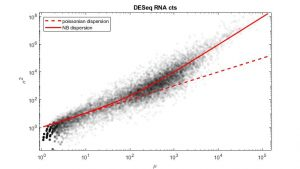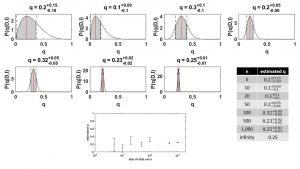# StatisticsHow do I know what the most appropriate statistical test for my data is?

What is multiple testing and why does it matter?

What should a histogram of p values look like, if H0 was true?

How do I measure the exact content of information in my data and how can I unveil information that lays dormant in the data without over interpreting it?

We are going to answer all these questions with hands-on examples by teaching not only the classical methods, but also more suitable tools like Bayesian Statistics.

Topics:

• probability
• basic probability density functions and their relations
• mean, variance and covariance
• t-test, z-test, ANOVA, MANOVA
• non-parametric tests
• multiple testing
• analyzing count data (peptides, DESeq)
• principal component analysis
• bayesian statisitcs